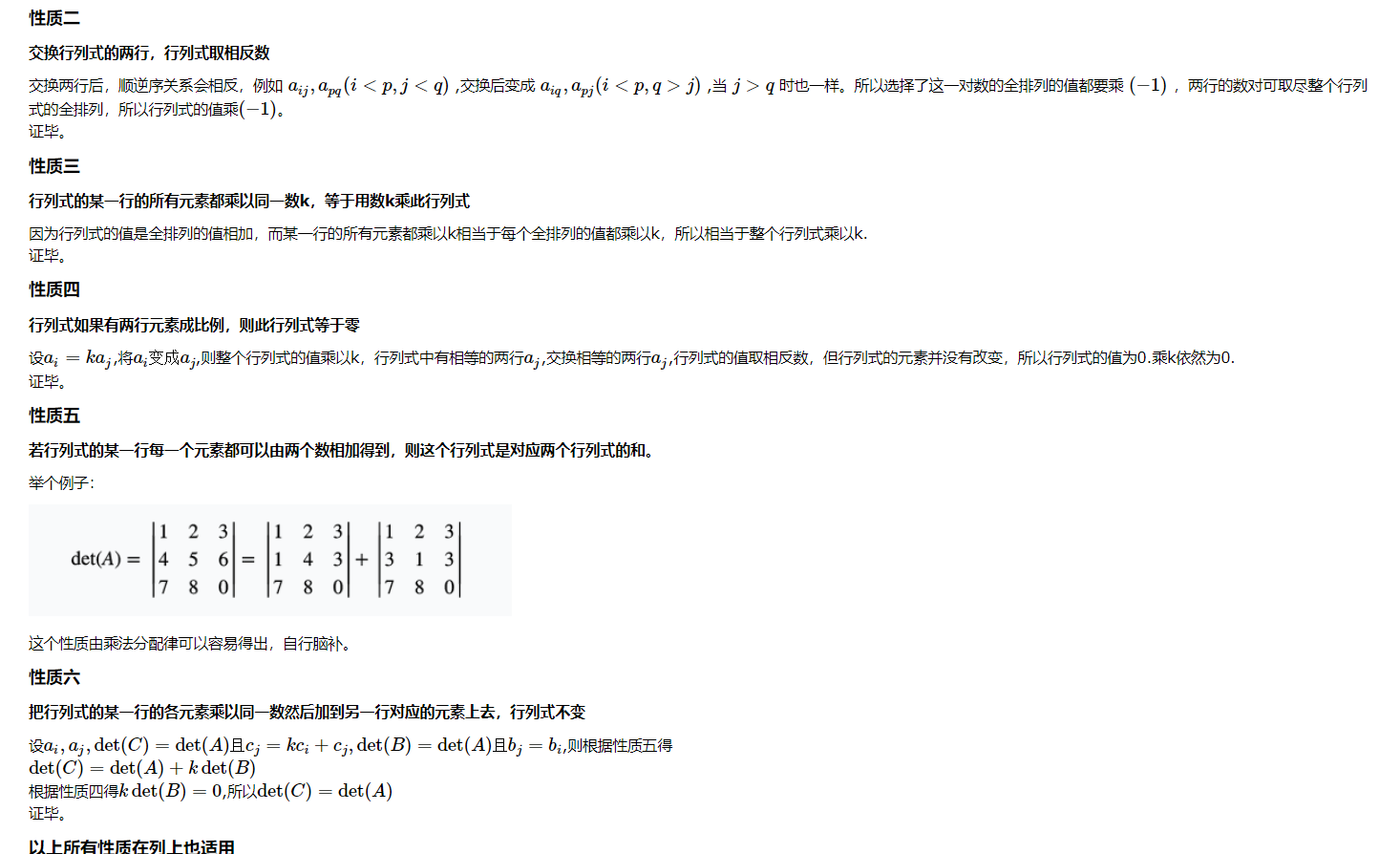# 高斯消元

## 行列式求解思路

1、高斯消元交换两行时答案要除以$(−1)$.(性质二)
2、假设处理到第$i$个方程，一般的高斯消元是用第$i$个方程减去第$j(j>i$)个方程,所得的答案作为新的第$j$个方程，但求行列式的时候要用第$j$行减去第$i$行，所得答案作为新的第$j$行。因为方式一相当于某一行乘$(−1)$,另一行加到这一行上，这并不符合性质六。